# GZ Curves (Part 2: Application)

Q. How does the slope of initial part of GZ curve represent the size of GM0? How is GM0 found from a GZ curve?
Ans. The initial part of the GZ curve is straight line rather than curvilinear. The slope of this part (up to 70) also represents the slope at origin.

In the figure θo is a small angle and S represents 57.3o. Triangle OPQ and ORS are similar triangles.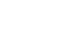………………………1

But at small angle θo, PQ = GZ at small angle = GMO Sin θ. But at small angle sin θ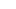θ we can say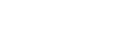…………………………..2

Substituting (2) in (1) we can say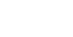=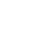Or RS = GMo.

Q. What is the effect of transverse shift of a weight on a GZ curve?
Ans. If a weight within the ship is shifted athwartship (// to deck), COG also will shift parallel and proportional to the shift.

This will cause reduction in righting lever on all angles of heel not equal to GG1 but equal to GG1 Cos θ. (GG, being equal to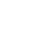).

This means the GZ value at 0o heel = 0 – GG1 Cos θ = – GG1. Thus, the righting lever of a listed ship in upright position is – GG1.

Q. What is the effect of vertical shift of a weight within the ship?
Ans. If a weight is shifted vertically upwards or downwards, the shift of COG will be parallel and proportional to w × d.  The shift of COG = GG1=.
The decrease / increase in the size of righting lever will be GG1 Sin θ for upward / downward shift respectively.

For the downward shift of weight, the GZ curve will increase in size vertically as well as horizontally.

Q. Can an upward shift of large consignment cause a negative GM? How do you explain this with the help of The GZ curve?
Ans. Yes, the initial or upright GM can become negative due to a shift of large consignment upwards, if initial GM was low prior shifting. The GZ value still remains zero at 0o heel but the subsequent values of resultant righting lever become negative implying that the vessel will not return to upright at slightest of disturbance. She may reach equilibrium again at some inclination θo. At θo, the GZ curve has an upward gradient showing that the GM at this equilibrium is positive. Also at this angle, i.e. angle of loll KN – KG Sin θ must equal GG1 Sin θ.

Q. How is the area under the GZ curve calculated till say angle of flooding say 38o?Ans. The vertical axis is righting lever (unit being metre). Horizontal axis is heel in degrees. The area is measured in metre radian, which means that the degrees must be converted to radians by dividing it with 57.3.

The range, 0o to 38o can be divided in 2 or 3 segments and area calculated by Simpson’s 1st or 2nd rule respectively. Thus, if the 1st rule is used then the value of uniform separation will beradians.

If the ordinates were 0, 0.12m and 0.29m, the area would be

Q. How is the GZ curve used to ensure that the vessel satisfies Severe Wind Criteria? Why is it necessary?
Ans. All cargo ships as well as passenger ships must show the satisfaction to severe wind criteria. A vessel might have the required intact stability criteria but may also have such windage area due freeboard, deck cargo, funnel, superstructure, etc that the ship’s behavior in nominal beam winds will heel her dangerously under steady and gusty wind impacts. A mirror inverted image of GZ curve is drawn on the left of the GZ curve for windward side. A steady wind heel lever is calculated as ,

where z is the vertical distance between COB & centre of windage and ∆ is the displacement in tonnes. 504 N/m2 is the nominal wind pressure used. A straight line // to x-axis is drawn at distance = lw1 from x-axis. Intersection of this line and GZ curve gives steady wind heel equilibrium angleo), which must be less than 16o or 80% of deck edge immersion angle (whichever is less). The lw2 is 1.5 × lw1. Even  lw2 is drawn // to x axis. θ1 is calculated using the information (4 tables) given in stability manual and measured from and to left of θo. Area on the leeward side (b) should be more than or equal to the area on windward side (a).

Q. Will the ship’s list change when she goes from one density to other?
Ans. The list will change when she goes from one density to other.

When a listed ship goes from one density to the other, GG1 does not change but KB and BM change. KB changes due to the change of draft and BM changes mainly due to change of underwater volume, the water plane area may be assumed to be constant.

Q. How do you justify this change with the help of GZ curve?
Ans. GZ curve values are derived from the equation KN – KG Sin θ. KN value for ship varies with inclination and underwater volume at that heel. Since, there is a change in underwater volume, KN value will change as the ship goes from one density to other. Though KN tables are given for different displacement values as argument (in SW), in a way they are related to the underwater volume. For a change in underwater volume KN value for a given heel changes causing the entire GZ curve to shift upwards or downwards, causing a shift of curve’s intersection point with x-axis and hence change in list.

Q. What is the influence of ship’s beam, freeboard and KG on GZ curve?
Ans. The form of ship above and below water both are important in deciding the size and shape of GZ curve. The 3 elements of form are draft, beam and free board.

If the beam is increased the curve’s starting phase becomes steeper. For a box vessel BMT =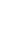. The GMO increases and therefore the GZ at various angles also increases. There is a change in maximum GZ value. Angle of vanishing stability also improves. (Curve B). We may also say that beam to draft ratio plays a big role in deciding if a ship in general will be tender or stiff.

With the increase in freeboard (instead of beam) the initial part of the curve remains same as original curve but the angle of vanishing stability shoots up. The GZ is a function of v × bb1. The increase of freeboard means the increase of v as well as bb1 till a larger angle, which is angle of deck edge immersion. After deck edge immersion, though v increases bb1 starts decreasing. (Curve C)

Thus, the increase of beam does not influence the size to GZ curve to that great an extent as can be done by increase in freeboard. The freeboard has tremendous effects on stability at moderate and large angles of heel. Since, the free board determines the angle of deck edge immersion and hence, the maximum values of stability parameters, the overall stability may also be considered as a function of free board. At the same time, it is worth nothing that freeboard has no effect on initial stability. From the condition C, if the COG is raised the GM and GZ are immediately affected. Range of positive stability reduces too. (Curve D)

(You may also visit my youtube videos @captsschaudhari.com)